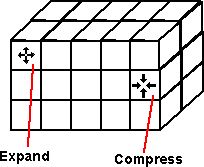# Physics - Deformation using Numerical Methods

We could divide an object into a finite number of parts, in 3 dimensions, possibly a 4th dimension for time, and against this calculate some physical properties? compression, inertia, etc.Can we model a solid object by dividing it up onto cubes (or some other regular shape). Then modeling the forces (or pressure) and the amount of compression or expansion in each cube.

One way to do this might be to keep the size of each cube constant, and to model the movement of mass between cubes as they expand or compress.

I'm not sure how we could handle different types of materials (example metal, plastic, foam, etc.). How do we handle the boundary between these materials? What if different material occupy an individual cube.

Should the position of cubes be absolute positions in space, or should each object be modeled separately relative to its position in the scenegraph? I cant see much point in using a scenegraph in this type of modeling, because quantities such as inertial all have to be modeled in the same frame of reference to produce realistic simulations.It seems quite difficult to model an object subject to bending forces. Is it realistic to expect to be able to model bending in this way, This could be a good test of this model.